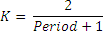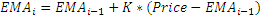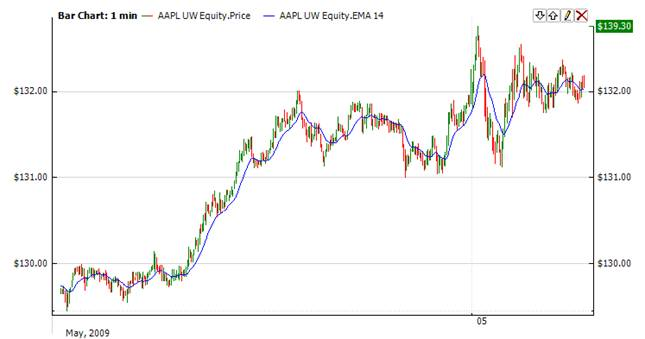﻿Exponential Moving Average

# Exponential Moving Average

The Exponential Moving Average (Ema) is a staple of technical analysis and is used in countless technical indicators.  In a Simple Moving Average, each value in the time period carries equal weight, and values outside of the time period are not included in the average.  However, the Exponential Moving Average is a cumulative calculation, including all data.  Past values have a diminishing contribution to the average, while more recent values have a greater contribution. This method allows the moving average to be more responsive to changes in the data.Market Signals

Typically, the signal to buy comes in when the Ema crosses price from above. When Ema crosses price from below, it is a signal to sell.CalculationChart ExampleImplementation and Usage

To initialize Exponential Moving Average indicator, use one of the following constructors:

Ema – set default values: period = 14

Ema(Int32) – set value for period

Ema(Double) – set value for factor (K in formula)

Use

EMA - property to get current valueExample
C#
``` 1// Create new instance
2Ema ema = new Ema(28);
3
4// Number of stored values
5ema.HistoryCapacity = 2;
6